# 4.4 Graphs of logarithmic functions  (Page 8/8)

 Page 8 / 8

## Verbal

The inverse of every logarithmic function is an exponential function and vice-versa. What does this tell us about the relationship between the coordinates of the points on the graphs of each?

Since the functions are inverses, their graphs are mirror images about the line $\text{\hspace{0.17em}}y=x.\text{\hspace{0.17em}}$ So for every point $\text{\hspace{0.17em}}\left(a,b\right)\text{\hspace{0.17em}}$ on the graph of a logarithmic function, there is a corresponding point $\text{\hspace{0.17em}}\left(b,a\right)\text{\hspace{0.17em}}$ on the graph of its inverse exponential function.

What type(s) of translation(s), if any, affect the range of a logarithmic function?

What type(s) of translation(s), if any, affect the domain of a logarithmic function?

Shifting the function right or left and reflecting the function about the y-axis will affect its domain.

Consider the general logarithmic function $\text{\hspace{0.17em}}f\left(x\right)={\mathrm{log}}_{b}\left(x\right).\text{\hspace{0.17em}}$ Why can’t $\text{\hspace{0.17em}}x\text{\hspace{0.17em}}$ be zero?

Does the graph of a general logarithmic function have a horizontal asymptote? Explain.

No. A horizontal asymptote would suggest a limit on the range, and the range of any logarithmic function in general form is all real numbers.

## Algebraic

For the following exercises, state the domain and range of the function.

$f\left(x\right)={\mathrm{log}}_{3}\left(x+4\right)$

$h\left(x\right)=\mathrm{ln}\left(\frac{1}{2}-x\right)$

Domain: $\text{\hspace{0.17em}}\left(-\infty ,\frac{1}{2}\right);\text{\hspace{0.17em}}$ Range: $\text{\hspace{0.17em}}\left(-\infty ,\infty \right)$

$g\left(x\right)={\mathrm{log}}_{5}\left(2x+9\right)-2$

$h\left(x\right)=\mathrm{ln}\left(4x+17\right)-5$

Domain: $\text{\hspace{0.17em}}\left(-\frac{17}{4},\infty \right);\text{\hspace{0.17em}}$ Range: $\text{\hspace{0.17em}}\left(-\infty ,\infty \right)$

$f\left(x\right)={\mathrm{log}}_{2}\left(12-3x\right)-3$

For the following exercises, state the domain and the vertical asymptote of the function.

$\text{\hspace{0.17em}}f\left(x\right)={\mathrm{log}}_{b}\left(x-5\right)$

Domain: $\text{\hspace{0.17em}}\left(5,\infty \right);\text{\hspace{0.17em}}$ Vertical asymptote: $\text{\hspace{0.17em}}x=5$

$\text{\hspace{0.17em}}g\left(x\right)=\mathrm{ln}\left(3-x\right)$

$\text{\hspace{0.17em}}f\left(x\right)=\mathrm{log}\left(3x+1\right)$

Domain: $\text{\hspace{0.17em}}\left(-\frac{1}{3},\infty \right);\text{\hspace{0.17em}}$ Vertical asymptote: $\text{\hspace{0.17em}}x=-\frac{1}{3}$

$\text{\hspace{0.17em}}f\left(x\right)=3\mathrm{log}\left(-x\right)+2$

$\text{\hspace{0.17em}}g\left(x\right)=-\mathrm{ln}\left(3x+9\right)-7$

Domain: $\text{\hspace{0.17em}}\left(-3,\infty \right);\text{\hspace{0.17em}}$ Vertical asymptote: $\text{\hspace{0.17em}}x=-3$

For the following exercises, state the domain, vertical asymptote, and end behavior of the function.

$f\left(x\right)=\mathrm{ln}\left(2-x\right)$

$f\left(x\right)=\mathrm{log}\left(x-\frac{3}{7}\right)$

Domain: $\left(\frac{3}{7},\infty \right)$ ;
Vertical asymptote: $x=\frac{3}{7}$ ; End behavior: as $x\to {\left(\frac{3}{7}\right)}^{+},f\left(x\right)\to -\infty$ and as $x\to \infty ,f\left(x\right)\to \infty$

$h\left(x\right)=-\mathrm{log}\left(3x-4\right)+3$

$g\left(x\right)=\mathrm{ln}\left(2x+6\right)-5$

Domain: $\left(-3,\infty \right)$ ; Vertical asymptote: $x=-3$ ;
End behavior: as $x\to -{3}^{+}$ , $f\left(x\right)\to -\infty$ and as $x\to \infty$ , $f\left(x\right)\to \infty$

$f\left(x\right)={\mathrm{log}}_{3}\left(15-5x\right)+6$

For the following exercises, state the domain, range, and x - and y -intercepts, if they exist. If they do not exist, write DNE.

$h\left(x\right)={\mathrm{log}}_{4}\left(x-1\right)+1$

Domain: $\text{\hspace{0.17em}}\left(1,\infty \right);\text{\hspace{0.17em}}$ Range: $\text{\hspace{0.17em}}\left(-\infty ,\infty \right);\text{\hspace{0.17em}}$ Vertical asymptote: $\text{\hspace{0.17em}}x=1;\text{\hspace{0.17em}}$ x -intercept: $\text{\hspace{0.17em}}\left(\frac{5}{4},0\right);\text{\hspace{0.17em}}$ y -intercept: DNE

$f\left(x\right)=\mathrm{log}\left(5x+10\right)+3$

$g\left(x\right)=\mathrm{ln}\left(-x\right)-2$

Domain: $\text{\hspace{0.17em}}\left(-\infty ,0\right);\text{\hspace{0.17em}}$ Range: $\text{\hspace{0.17em}}\left(-\infty ,\infty \right);\text{\hspace{0.17em}}$ Vertical asymptote: $\text{\hspace{0.17em}}x=0;\text{\hspace{0.17em}}$ x -intercept: $\text{\hspace{0.17em}}\left(-{e}^{2},0\right);\text{\hspace{0.17em}}$ y -intercept: DNE

$f\left(x\right)={\mathrm{log}}_{2}\left(x+2\right)-5$

$h\left(x\right)=3\mathrm{ln}\left(x\right)-9$

Domain: $\text{\hspace{0.17em}}\left(0,\infty \right);\text{\hspace{0.17em}}$ Range: $\text{\hspace{0.17em}}\left(-\infty ,\infty \right);\text{\hspace{0.17em}}$ Vertical asymptote: $\text{\hspace{0.17em}}x=0;\text{\hspace{0.17em}}$ x -intercept: $\text{\hspace{0.17em}}\left({e}^{3},0\right);\text{\hspace{0.17em}}$ y -intercept: DNE

## Graphical

For the following exercises, match each function in [link] with the letter corresponding to its graph.

$d\left(x\right)=\mathrm{log}\left(x\right)$

$f\left(x\right)=\mathrm{ln}\left(x\right)$

B

$g\left(x\right)={\mathrm{log}}_{2}\left(x\right)$

$h\left(x\right)={\mathrm{log}}_{5}\left(x\right)$

C

$j\left(x\right)={\mathrm{log}}_{25}\left(x\right)$

For the following exercises, match each function in [link] with the letter corresponding to its graph.

$f\left(x\right)={\mathrm{log}}_{\frac{1}{3}}\left(x\right)$

B

$g\left(x\right)={\mathrm{log}}_{2}\left(x\right)$

$h\left(x\right)={\mathrm{log}}_{\frac{3}{4}}\left(x\right)$

C

For the following exercises, sketch the graphs of each pair of functions on the same axis.

$f\left(x\right)=\mathrm{log}\left(x\right)\text{\hspace{0.17em}}$ and $\text{\hspace{0.17em}}g\left(x\right)={10}^{x}$

$f\left(x\right)=\mathrm{log}\left(x\right)\text{\hspace{0.17em}}$ and $\text{\hspace{0.17em}}g\left(x\right)={\mathrm{log}}_{\frac{1}{2}}\left(x\right)$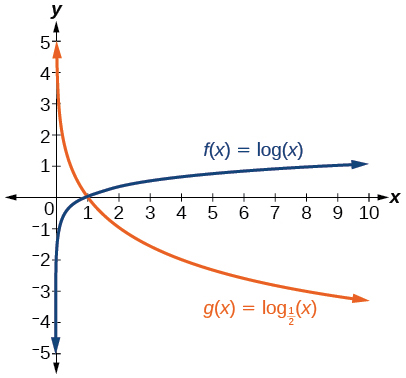$f\left(x\right)={\mathrm{log}}_{4}\left(x\right)\text{\hspace{0.17em}}$ and $\text{\hspace{0.17em}}g\left(x\right)=\mathrm{ln}\left(x\right)$

$f\left(x\right)={e}^{x}\text{\hspace{0.17em}}$ and $\text{\hspace{0.17em}}g\left(x\right)=\mathrm{ln}\left(x\right)$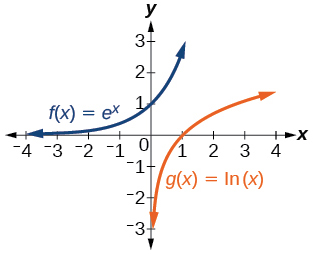For the following exercises, match each function in [link] with the letter corresponding to its graph.

$f\left(x\right)={\mathrm{log}}_{4}\left(-x+2\right)$

$g\left(x\right)=-{\mathrm{log}}_{4}\left(x+2\right)$

C

$h\left(x\right)={\mathrm{log}}_{4}\left(x+2\right)$

For the following exercises, sketch the graph of the indicated function.

$\text{\hspace{0.17em}}f\left(x\right)={\mathrm{log}}_{2}\left(x+2\right)$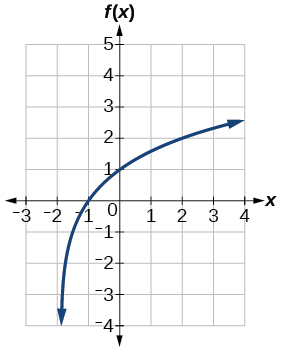$\text{\hspace{0.17em}}f\left(x\right)=2\mathrm{log}\left(x\right)$

$\text{\hspace{0.17em}}f\left(x\right)=\mathrm{ln}\left(-x\right)$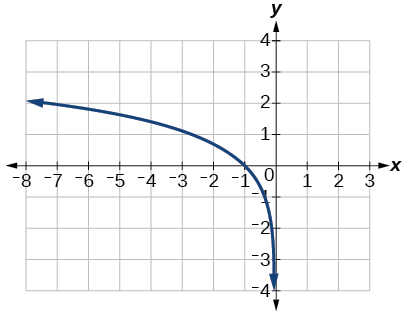$g\left(x\right)=\mathrm{log}\left(4x+16\right)+4$

$g\left(x\right)=\mathrm{log}\left(6-3x\right)+1$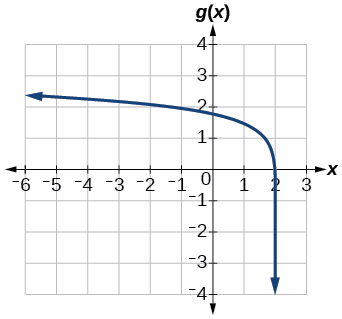$h\left(x\right)=-\frac{1}{2}\mathrm{ln}\left(x+1\right)-3$

For the following exercises, write a logarithmic equation corresponding to the graph shown.

Use $\text{\hspace{0.17em}}y={\mathrm{log}}_{2}\left(x\right)\text{\hspace{0.17em}}$ as the parent function.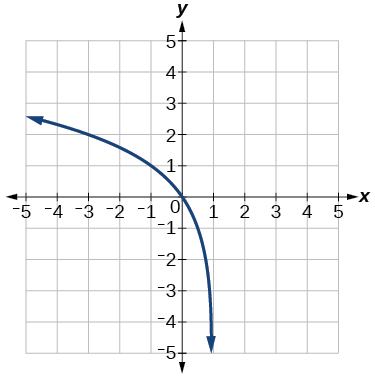$\text{\hspace{0.17em}}f\left(x\right)={\mathrm{log}}_{2}\left(-\left(x-1\right)\right)$

Use $\text{\hspace{0.17em}}f\left(x\right)={\mathrm{log}}_{3}\left(x\right)\text{\hspace{0.17em}}$ as the parent function.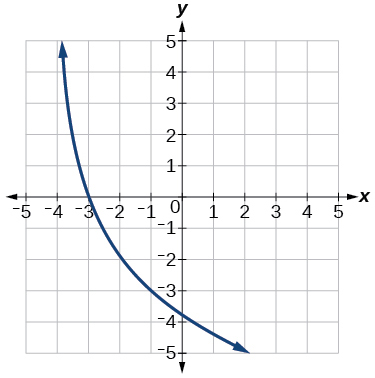Use $\text{\hspace{0.17em}}f\left(x\right)={\mathrm{log}}_{4}\left(x\right)\text{\hspace{0.17em}}$ as the parent function.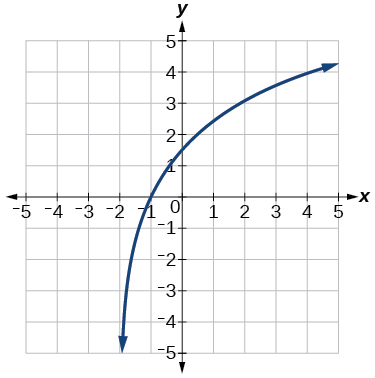$f\left(x\right)=3{\mathrm{log}}_{4}\left(x+2\right)$

Use $\text{\hspace{0.17em}}f\left(x\right)={\mathrm{log}}_{5}\left(x\right)\text{\hspace{0.17em}}$ as the parent function.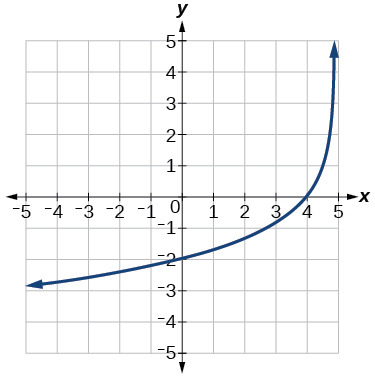## Technology

For the following exercises, use a graphing calculator to find approximate solutions to each equation.

$\mathrm{log}\left(x-1\right)+2=\mathrm{ln}\left(x-1\right)+2$

$x=2$

$\mathrm{log}\left(2x-3\right)+2=-\mathrm{log}\left(2x-3\right)+5$

$\mathrm{ln}\left(x-2\right)=-\mathrm{ln}\left(x+1\right)$

$x\approx \text{2}\text{.303}$

$2\mathrm{ln}\left(5x+1\right)=\frac{1}{2}\mathrm{ln}\left(-5x\right)+1$

$\frac{1}{3}\mathrm{log}\left(1-x\right)=\mathrm{log}\left(x+1\right)+\frac{1}{3}$

$x\approx -0.472$

## Extensions

Let $\text{\hspace{0.17em}}b\text{\hspace{0.17em}}$ be any positive real number such that $\text{\hspace{0.17em}}b\ne 1.\text{\hspace{0.17em}}$ What must $\text{\hspace{0.17em}}{\mathrm{log}}_{b}1\text{\hspace{0.17em}}$ be equal to? Verify the result.

Explore and discuss the graphs of $\text{\hspace{0.17em}}f\left(x\right)={\mathrm{log}}_{\frac{1}{2}}\left(x\right)\text{\hspace{0.17em}}$ and $\text{\hspace{0.17em}}g\left(x\right)=-{\mathrm{log}}_{2}\left(x\right).\text{\hspace{0.17em}}$ Make a conjecture based on the result.

The graphs of $\text{\hspace{0.17em}}f\left(x\right)={\mathrm{log}}_{\frac{1}{2}}\left(x\right)\text{\hspace{0.17em}}$ and $\text{\hspace{0.17em}}g\left(x\right)=-{\mathrm{log}}_{2}\left(x\right)\text{\hspace{0.17em}}$ appear to be the same; Conjecture: for any positive base $\text{\hspace{0.17em}}b\ne 1,$ $\text{\hspace{0.17em}}{\mathrm{log}}_{b}\left(x\right)=-{\mathrm{log}}_{\frac{1}{b}}\left(x\right).$

Prove the conjecture made in the previous exercise.

What is the domain of the function $\text{\hspace{0.17em}}f\left(x\right)=\mathrm{ln}\left(\frac{x+2}{x-4}\right)?\text{\hspace{0.17em}}$ Discuss the result.

Recall that the argument of a logarithmic function must be positive, so we determine where $\text{\hspace{0.17em}}\frac{x+2}{x-4}>0\text{\hspace{0.17em}}$ . From the graph of the function $\text{\hspace{0.17em}}f\left(x\right)=\frac{x+2}{x-4},$ note that the graph lies above the x -axis on the interval $\text{\hspace{0.17em}}\left(-\infty ,-2\right)\text{\hspace{0.17em}}$ and again to the right of the vertical asymptote, that is $\text{\hspace{0.17em}}\left(4,\infty \right).\text{\hspace{0.17em}}$ Therefore, the domain is $\text{\hspace{0.17em}}\left(-\infty ,-2\right)\cup \left(4,\infty \right).$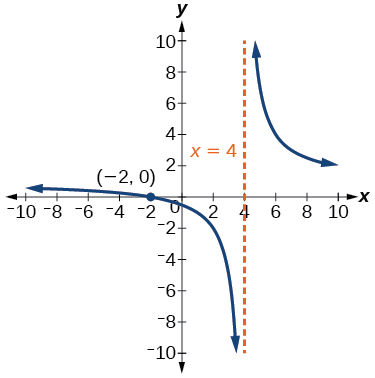Use properties of exponents to find the x -intercepts of the function $\text{\hspace{0.17em}}f\left(x\right)=\mathrm{log}\left({x}^{2}+4x+4\right)\text{\hspace{0.17em}}$ algebraically. Show the steps for solving, and then verify the result by graphing the function.

#### Questions & Answers

x=-b+_Гb2-(4ac) ______________ 2a
I've run into this: x = r*cos(angle1 + angle2) Which expands to: x = r(cos(angle1)*cos(angle2) - sin(angle1)*sin(angle2)) The r value confuses me here, because distributing it makes: (r*cos(angle2))(cos(angle1) - (r*sin(angle2))(sin(angle1)) How does this make sense? Why does the r distribute once
so good
abdikarin
this is an identity when 2 adding two angles within a cosine. it's called the cosine sum formula. there is also a different formula when cosine has an angle minus another angle it's called the sum and difference formulas and they are under any list of trig identities
How can you tell what type of parent function a graph is ?
generally by how the graph looks and understanding what the base parent functions look like and perform on a graph
William
if you have a graphed line, you can have an idea by how the directions of the line turns, i.e. negative, positive, zero
William
y=x will obviously be a straight line with a zero slope
William
y=x^2 will have a parabolic line opening to positive infinity on both sides of the y axis vice versa with y=-x^2 you'll have both ends of the parabolic line pointing downward heading to negative infinity on both sides of the y axis
William
y=x will be a straight line, but it will have a slope of one. Remember, if y=1 then x=1, so for every unit you rise you move over positively one unit. To get a straight line with a slope of 0, set y=1 or any integer.
Aaron
yes, correction on my end, I meant slope of 1 instead of slope of 0
William
what is f(x)=
I don't understand
Joe
Typically a function 'f' will take 'x' as input, and produce 'y' as output. As 'f(x)=y'. According to Google, "The range of a function is the complete set of all possible resulting values of the dependent variable (y, usually), after we have substituted the domain."
Thomas
Sorry, I don't know where the "Â"s came from. They shouldn't be there. Just ignore them. :-)
Thomas
Darius
Thanks.
Thomas
Â
Thomas
It is the Â that should not be there. It doesn't seem to show if encloses in quotation marks. "Â" or 'Â' ... Â
Thomas
Now it shows, go figure?
Thomas
what is this?
i do not understand anything
unknown
lol...it gets better
Darius
I've been struggling so much through all of this. my final is in four weeks 😭
Tiffany
this book is an excellent resource! have you guys ever looked at the online tutoring? there's one that is called "That Tutor Guy" and he goes over a lot of the concepts
Darius
thank you I have heard of him. I should check him out.
Tiffany
is there any question in particular?
Joe
I have always struggled with math. I get lost really easy, if you have any advice for that, it would help tremendously.
Tiffany
Sure, are you in high school or college?
Darius
Hi, apologies for the delayed response. I'm in college.
Tiffany
how to solve polynomial using a calculator
So a horizontal compression by factor of 1/2 is the same as a horizontal stretch by a factor of 2, right?
The center is at (3,4) a focus is at (3,-1), and the lenght of the major axis is 26
The center is at (3,4) a focus is at (3,-1) and the lenght of the major axis is 26 what will be the answer?
Rima
I done know
Joe
What kind of answer is that😑?
Rima
I had just woken up when i got this message
Joe
Can you please help me. Tomorrow is the deadline of my assignment then I don't know how to solve that
Rima
i have a question.
Abdul
how do you find the real and complex roots of a polynomial?
Abdul
@abdul with delta maybe which is b(square)-4ac=result then the 1st root -b-radical delta over 2a and the 2nd root -b+radical delta over 2a. I am not sure if this was your question but check it up
Nare
This is the actual question: Find all roots(real and complex) of the polynomial f(x)=6x^3 + x^2 - 4x + 1
Abdul
@Nare please let me know if you can solve it.
Abdul
I have a question
juweeriya
hello guys I'm new here? will you happy with me
mustapha
The average annual population increase of a pack of wolves is 25.
how do you find the period of a sine graph
Period =2π if there is a coefficient (b), just divide the coefficient by 2π to get the new period
Am
if not then how would I find it from a graph
Imani
by looking at the graph, find the distance between two consecutive maximum points (the highest points of the wave). so if the top of one wave is at point A (1,2) and the next top of the wave is at point B (6,2), then the period is 5, the difference of the x-coordinates.
Am
you could also do it with two consecutive minimum points or x-intercepts
Am
I will try that thank u
Imani
Case of Equilateral Hyperbola
ok
Zander
ok
Shella
f(x)=4x+2, find f(3)
Benetta
f(3)=4(3)+2 f(3)=14
lamoussa
14
Vedant
pre calc teacher: "Plug in Plug in...smell's good" f(x)=14
Devante
8x=40
Chris
Explain why log a x is not defined for a < 0
the sum of any two linear polynomial is what
divide simplify each answer 3/2÷5/4
divide simplify each answer 25/3÷5/12
Momo
how can are find the domain and range of a relations
the range is twice of the natural number which is the domain
MorolakeByByBy Anindyo MukhopadhyayBy Mariah HauptmanByBy Mistry BhaveshByBy